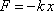Force of spring EQUALS minus k TIMES x

In magnitude, the force F exerted by a spring on an object "attached" to the end of the spring (in the case of a compressed spring it is sufficient that the object be in contact with the spring) is equal to the product of the spring constant k (a.k.a. the force constant of the spring) times the amount of stretch or compression x of the spring.

Note: The minus sign is part of a typically unnecessary algebraic sign convention used for keeping track of the direction of the force. In cases involving a spring which is either stretched or compressed a fixed amount or undergoing a process in which the spring is varying in amount of stretch or compression but still either always stretched or always compressed, it is best to define the positive direction of the spring force by means of a diagram as the direction in which the force actually acts, and to assign that force the magnitude kx, rather than to worry about the minus sign in this equation.

 Pitfall Avoidance Notes: 1. The quantity x is not the length of the spring. It is the position of the object with respect to its equilibrium position where the equilibrium position is the position when the spring is neither stretched nor compressed. 2. The quantity k is not the force exerted by the spring. It is a characteristic of the spring which helps determine the force, but the force itself, in magnitude is k times x, not k.